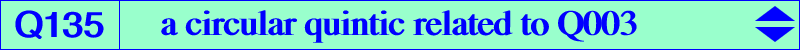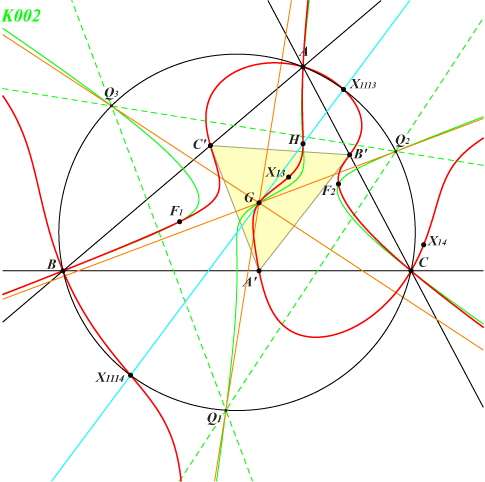too complicated to be written here. Click on the link to download a text file.X(2), X(4), X(13), X(14), X(1113), X(1114) infinite points of K002 foci of the Steiner inellipse vertices of the pedal triangle of X(2)Q003 and the union of the line at infinity, the circumcircle, the Kiepert hyperbola generate a pencil of circular trinodal circum-quintics which contains Q050, Q135 and several other curves. Each quintic of the pencil passes through A, B, C (which are nodes on the curve), X(2), X(4), X(13), X(14), X(1113), X(1114), the circular points at infinity (with tangents passing through X(2), the singular focus). The three real asymptotes are parallel to those of the Thomson cubic K002. Q(M) also contains the vertices A', B', C' of the pedal triangle of M. Hence, a quintic Q(M) of the pencil is entirely characterized by its fifth point M on the Euler line. With M = X(30), the quintic decomposes as said above. Since Q(M) has already ten known common points with the circumcircle, it must also decompose with M = X(1113) or X(1114). With M = X(4), we obtain Q003, tangent at X(4) to the Euler line and with M = X(2), we obtain Q135, tangent at X(2) to the Euler line. Q135 is the only proper quintic of the pencil having five asymptotes concurring at X(2). Two are isotropic and three are the altitudes of the Thomson triangle Q1Q2Q3. The isogonal transform of Q135 is Q137.